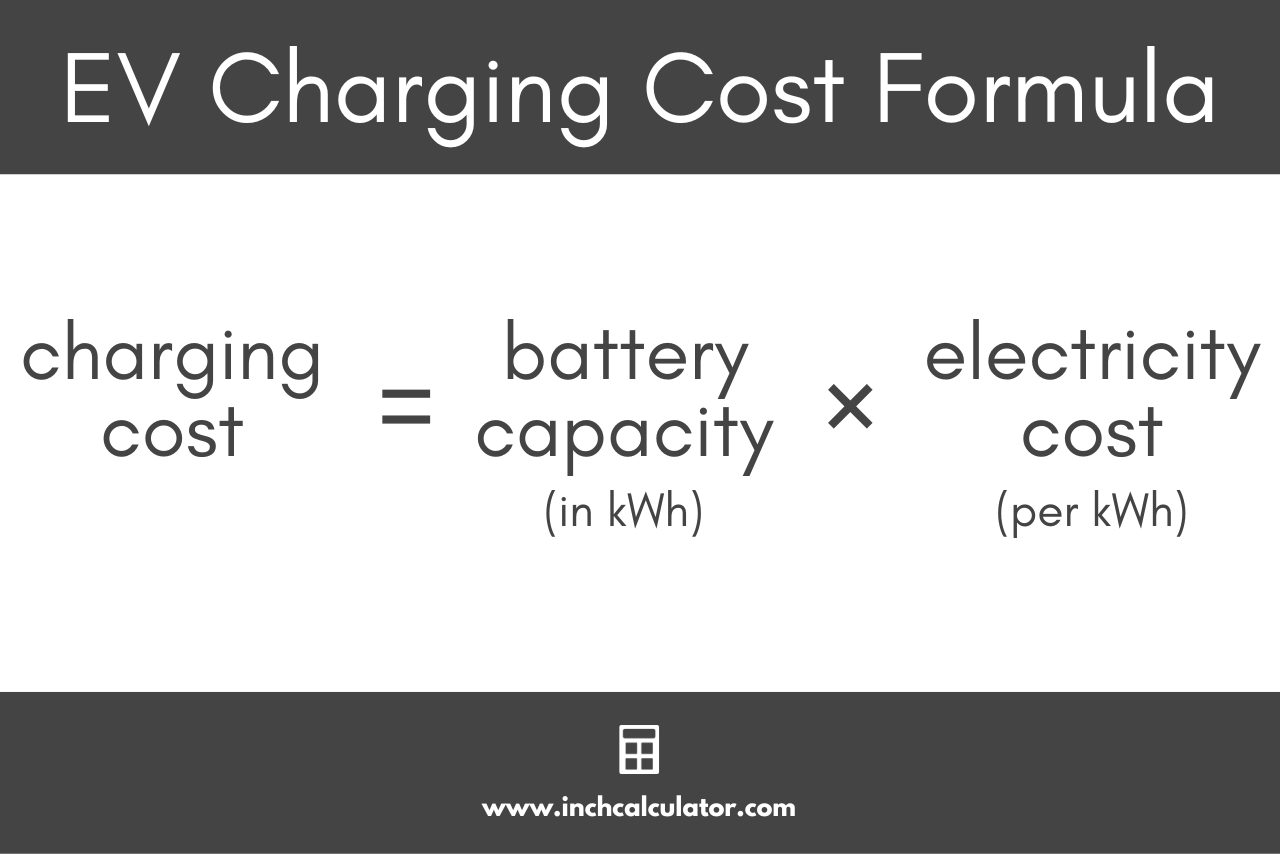# Electric Vehicle Charging Cost Calculator

Calculate how much it costs to charge an electric car from to full capacity or to drive a specific distance by entering the electricity cost and capacity below.

## Calculate the Cost to Charge to Capacity

kWh
per kWh
Note: the average price of electricity is about 14 cents per kWh.

## Calculate the Cost to Drive a Set Distance

per kWh
Note: the average price of electricity is about 14 cents per kWh.

## Electricity Cost Estimate:

\$
Learn how we calculated this below

## How to Estimate the Cost to Charge an Electric Car

Most cars today consume fossil fuels like gasoline, diesel, and compressed natural gas. When fossil fuels are burned, they release a ton of CO2, exacerbating the global environment. In comparison, electricity can be produced and transported easily and locally, making it much cheaper than any other fuel or energy medium. Electric vehicles use this fact to present themselves as an environmentally friendly alternative to fossil fuel cars and a cheaper one too.

Electric vehicles are appealing for their fuel cost savings, but estimating just how much an electric vehicle costs to charge can be a bit tricky. Fortunately, there are some easy formulas that can be used to calculate the cost to charge an electric car.

You can calculate how much it costs to charge a vehicle battery from empty to full capacity by multiplying the capacity of the battery in kilowatt-hours by the electricity cost per kilowatt-hour.

### Electric Vehicle Charging Cost Formula

Here’s the formula to calculate the cost to charge an electric car from empty to full:

charging cost = battery capacity(in kWh) × electricity cost(per kWh)

The cost of energy varies, but on average electricity rate is 13.87 cents per kilowatt-hour.. You can find the cost of energy on your electric bill or by using our electricity cost calculator.For example, let’s find the cost to charge a Tesla Model S, which has a 100 kWh battery to capacity.

charging cost = 100kWh × \$0.1387
charging cost = \$13.87 per full charge

Thus, it will cost about \$13.87 to charge a Tesla Model S to full capacity.

## Average Battery Charging Costs

The chart below shows the average cost to charge an electric vehicle to capacity for various battery sizes.

Table showing the average cost to charge an electric vehicle based on the national average electricity rate of \$0.1387 per kWh
Battery Capacity (kWh) Average Charge Cost
5 kWh \$0.69
10 kWh \$1.39
15 kWh \$2.08
20 kWh \$2.77
25 kWh \$3.47
30 kWh \$4.16
35 kWh \$4.85
40 kWh \$5.55
45 kWh \$6.24
50 kWh \$6.94
55 kWh \$7.63
60 kWh \$8.32
65 kWh \$9.02
70 kWh \$9.71
75 kWh \$10.40
80 kWh \$11.10
85 kWh \$11.79
90 kWh \$12.48
95 kWh \$13.18
100 kWh \$13.87
110 kWh \$15.26
120 kWh \$16.64
130 kWh \$18.03
140 kWh \$19.42
150 kWh \$20.81

You’ll probably also be interested in our charging time calculator.

## References

1. U.S. Energy Information Administration, During 2021, U.S. retail electricity prices rose at fastest rate since 2008, March 1, 2022, https://www.eia.gov/todayinenergy/detail.php?id=51438
2. U.S. Energy Information Administration, Table 5.6.A. Average Price of Electricity to Ultimate Customers by End-Use Sector, July 2022, https://www.eia.gov/electricity/monthly/epm_table_grapher.php?t=epmt_5_6_a
3. Edmunds, 2022 Tesla Model S, https://www.edmunds.com/tesla/model-s/